Models of Computation

# 13. Models of Computation

The first module of this course deals with the technical foundations of all digital systems, specialized to an endless variety of specific applications. For the remainder of the course, we refocus our attention on a particular class of digital system: the general purpose computer. The distinguishing feature of this class of digital systems is that their behavior is dictated by algorithms encoded as digital data, rather than being wired into the digital circuitry that constitutes their hardware design. This distinction represents a major conceptual revolution of the early $20^{th}$ century, and one that underlies modern computer science and engineering. Its immediate consequences are the conceptual distinction between hardware and software, the notion of programming and programming languages, interpretation and compilation as engineering techniques, and many other issues central to contemporary computer science. Fundamental ideas that underly this important paradigm shift are the topic of this Chapter. We may view these seminal ideas as the product of a search, during the first half of the twentieth century, for a suitable formal model of digital computation: a sound mathematical basis for the study of the universe of computational algorithms and machines capable of performing them.

This Chapter marks our transition to this new focus by sketching foundational ideas of Computer Science resulting from that search.

FSMs as a model of computation

## 13.1. FSMs as a model of computation

Finite state machines, described in Chapter 9, are an attractive candidate as a model of computation. We may view a simple ROM-based FSM as a programmable general-purpose (or at least multi-purpose) computation machine, in that its behavior may be modified simply by changing the string of bits stored in its ROM. Of course, any particular FSM implementation as a digital circuit necessarily fixes the number of inputs, outputs, and states as implementation constants, limiting the range of FSM behaviors that can be achieved by reprogramming its ROM.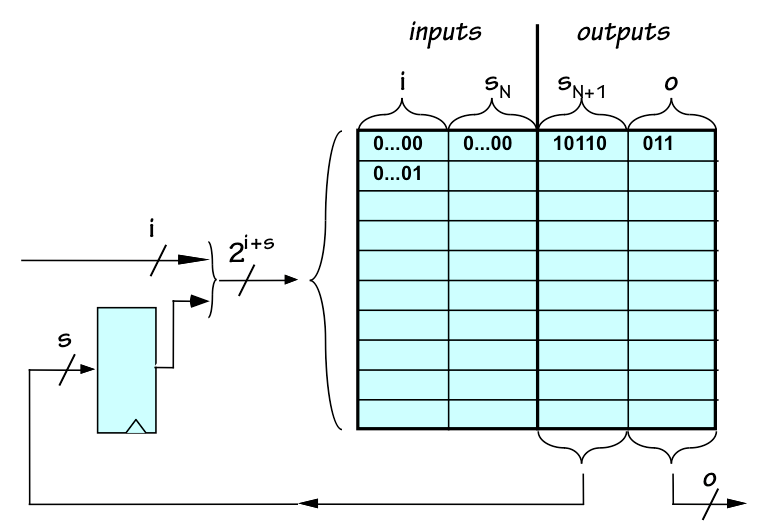Generalized FSM implementation
We might generalize our FSM model a bit by parameterizing the implementation as shown to the right. This design shows the implementation of an FSM with $i$ inputs, $o$ outputs, and $s$ state bits (for a maximum of $2^s$ states). Given an arbitrary FSM specification (for example, in the form of a state transition diagram) we can implement that FSM according to this diagram by an appropriate choice of the parameters $i$, $o$, and $s$ as well as the specific bits to be stored in the ROM. This is a useful conceptual tool, as it allows us characterize the universe of computations that can be performed by any FSM of any size as some instance of this diagram with concrete values for $i$, $o$, $s$, and ROM contents.

We might ask how many different FSM behaviors may be encoded into the ROM of this generalized FSM circuit for a given set of $i$, $o$, and $s$ values. Although this question is hard to answer precisely, an easy upper bound is the number of distinct ways in which the ROM of the given FSM can be programmed. Given the $i$, $o$, and $s$ parameters we can deduce that the number of lines in the ROM's truth table is $2^{i+s}$, as the ROM has $i+s$ inputs. Each line specifies $o+s$ output values; thus there are $(o+s)\cdot2^{i+s}$ binary output values to be specified for the ROM's truth table. since we may choose a 1 or zero for each, the total number of distinct ROM contents are $$FSMS(i, o, s) = 2^{(o+s)\cdot2^{i+s}}$$ The reason that this number is an upper bound rather than a precise count of distinct FSMs is that some pairs of distinct ROM contents yield equivalent FSMs. However, we are assured that any given FSM having $i$ inputs, $o$ outputs, and $s$ state bits will be equivalent to at least one of the FSM behaviors represented among the set of ROM programs considered.

FSM enumeration

### 13.1.1. FSM enumeration

Although the bound established by $FSMS(i,o,s)$ grows large as the parameters $i, o, s$ increase, we note that it remains finite for finite $i, o, s$. This observation suggests an intriguing conceptual exercise: we could define an infinite list that includes every possible FSM -- with some duplicates, due to equivalence -- by enumerating the possible ROM configurations for each set of $i, o, s$ values in some canonical order.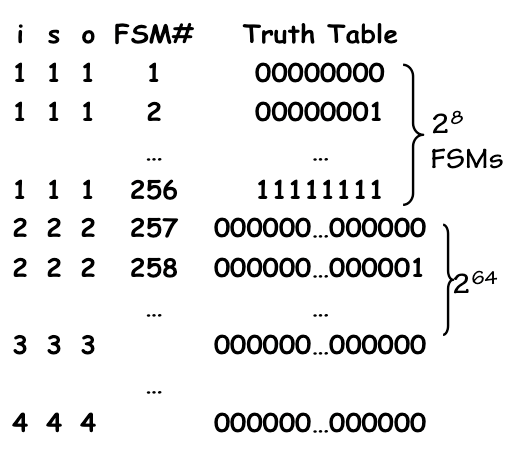FSM Enumeration
One approach to building such a list is sketched to the left. The first FSMs on the list are those with $i=o=s=1$. Each of these is programmed by specifying an 8-bit string as its ROM contents, so there are $2^8=256$ possible ROM configurations for this FSM size. The first 256 entries denote FSMs with $i=o=s=1$, whose 8-bit ROM contents are listed in natural binary order. Next on the list is FSMs having $i=o=s=2$; there are $FSMS(2,2,2) = 2^{64}$ of these, again added to the list by stepping through possible ROM contents in natural binary order. We then add FSMs having $i=o=s=3$, $i=o=s=4$, and so on for every finite value for $i=o=s$.

This construction lists all FSMs for which $i=o=s$, but seems to miss FSMs having different numbers of inputs, outputs, and state bits. Where on our list, for example, is an FSM having 1 input, 2 outputs, and 3 state bits? We justify such omissions by the observation that there is an equivalent FSM on the list with $i=o=s=3$ that behaves identically if we ignore unused inputs, outputs, or state bits. Our conceptual list, therefore, captures every possible FSM behavior at some finite position on the list.

Although there are many different schemes we could use to define our FSM enumeration, once we agree on an enumeration we can refer to the $i^{th}$ FSM, $FSM_i$, unambiguously. There becomes an effective procedure by which we can take any well-specified FSM and determine an index $i$ on our list for which $FSM_i$ behaves identically. We might discover that the 5-state ant controller of Section 9.4 is $FSM_{23785}$, and that the 4-state equivalent version of Section 9.4.1 is $FSM_{1823}$ (its earlier position on the list reflecting its reduced complexity).

Indeed, the indices of complex FSMs (like microprocessor chips) would quickly become huge. Each such FSM index, after all, encodes all of the information necessary to implement that FSM.

FSMs as a Model of Computation

### 13.1.2. FSMs as a Model of Computation

The ability to conceptually catalog FSMs of all sizes, and consequently the set of computations that can be performed by FSMs of all sizes, makes FSMs attractive as a formal model for the computations we might perform by digital circuitry. Moreover, FSMs have been extensively studied and their formal properties and capabilities are well understood. For example, for every class of strings described by a regular expression -- a parameterized template including "wild card" and repetition operators -- there is an FSM that will identify strings of that class. For these reasons, finite state machines have become, and remain, an important formal model for a class of computations.

A natural question arises as to whether FSMs are an appropriate formal model for all computations we might want to perform using digital circuitry. If every computation we might want a digital system to perform can be performed by some finite state machine, does this reduce the process of digital system engineering to the simple selection of an FSM from our enumerated catalog of possibilities? Of course, this extreme view ignores practical issues like cost/performance tradeoffs; however, the question of whether the class of computations performed by FSMs is a suitable abstraction for all practical digital computations is clearly worth answering.

FSM limitations

### 13.1.3. FSM limitations

Although FSMs can clearly perform a variety of useful operations, there are simple, well-defined computations that cannot be performed by any finite state machine.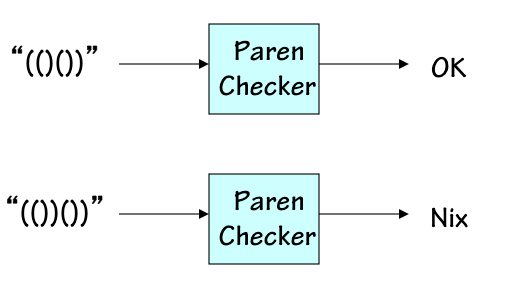Paren Checker
To take a simple example, imagine a device whose input is a sequence of left and right parentheses (perhaps encoded as 0 and 1, respectively) and whose single digital output is 1 if and only if the string entered thus far is a well formed parenthesis string. This test requires that each left paren in the string is matched by a subsequent right paren, perhaps separated by one or more well-formed paren strings. The skeptical reader is invited to devise an FSM that meets this specification, armed with the friendly warning that the challenge is a fool's errand.

The difficulty arises because of the finiteness of an FSM's storage. If an input string consisting of $k$ consecutive left parens is entered, the paren checker must use at least $k+1$ different states to count the number of left parens entered, since it can only output a $1$ if precisely $k$ right parens are subsequently entered. Thus at least $k+1$ states are required to respond properly to an input string consisting of $k$ left parens followed by $k$ right parens. For no finite $N$ can an $N$-state FSM properly deal with all possible finite inputs.

In fairness to FSMs as a model, the finiteness of their storage capacity is a deliberate design feature. As practical engineers, we are interested in computations that can be completed using finite resourses -- storage as well as time -- and FSMs as a class seem to capture this set of computations. Any specific FSM, however, offers only a bounded amount of storage for the computations it performs; hence no single FSM can perform a range of computations whose storage requirements are unbounded. Paren checking of arbitrary finite strings is an example: while any finite string can be checked using finite storage, for every finite bound we can find a finite input string whose storage requirements will exceed this bound. There are, of course, many other simple examples of practical algorithms we may wish to consider which require unbounded storage depending on their input data.

Alternative Models

### 13.1.4. Alternative Models

The search for an abstract model of computation capable of dealing with a broader class of algorithms was an active research topic during the early $20^{th}$ century. Proposed candidates explored a wide variety of approaches and characteristics, and many have had lasting impact on the field of computer science. Examples include:
• The lambda calculus of Alonso Church, simple model for functional programming languages;
• The combinatory logic of Schonfinkel and Curry, a model for functional programming without variables;
• The theory of recursive functions of Kleene;
• The string rewriting systems of Post and others, in which system state stored as a string of symbols evolves under a set of rewrite rules;
• Turing machines, discussed in the next section.
Each of these models aimed to capture a broad set of practical algorithms, including those whose time and space requirements were unbounded functions of their input data.

As new formalisms were proposed and compared, there was a clear need for a benchmark to determine their relative power as a general model of computation. What emerged as a standard measure of a model's power is the set of integer functions supported by that model: a model that could support addition and multiplication of arbitrary integers, for example, was more powerful than an alternative model that could add but not multiply.

Turing Machines

## 13.2. Turing Machines

One of the alternative formalisms for modeling computation was a simple extension of FSMs proposed by Alan Turing in 1936.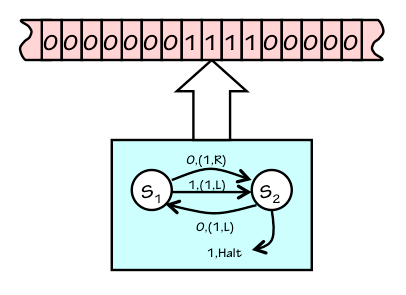A Turing Machine
Turing addressed the bounded storage limitation of the FSM by a simple expedient: a Turing Machine is a finite state machine interfaced to a doubly infinite digital tape (meaning a tape with neither a left nor a right end). A handful of the FSM's inputs and outputs are used to control the tape interface, which consists of a tape head pointing to some discrete position on the tape and the ability o read a binary digit (1 or 0) at the current position, write a binary digit at the current position, and/or move the head one position to the left or right on the tape.

The infinite tape of a Turing Machine serves as its sole input/output device: the FSM inputs and outputs serve only to interface to the tape. A computation is performed on a Turing Machine by

• Mounting a tape containing some initial configuration of 0s and 1s, with the tape head pointing to a appropriate starting point. This configuration encodes an input to the computation.
• Starting the Turing Machine (with its FSM in a designated inital state).
• Letting the Turing Machine run until its FSM reaches a special halting state.
• Reading the final configuration of 1s and 0s from the tape of the halted machine. This configuration encodes the output of the computation.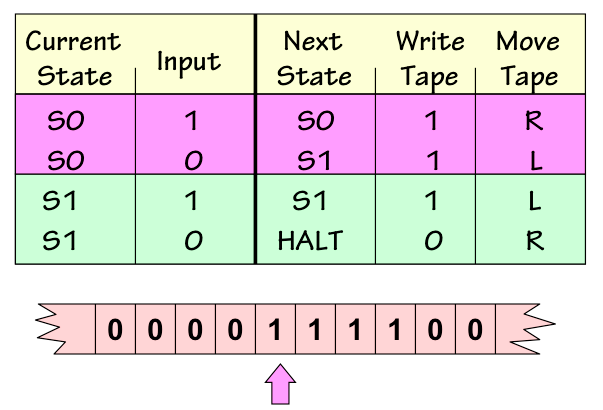Unary Incrementor TM
A simple Turing machine example is shown in the diagram to the right. This machine consists of a 2-state FSM, and is shown with an initial tape configuration consisting of a sequence of four 1s starting at the current head position. This tape configuration encodes the value 4 in a primitive unary format, in which a nonnegative integer $n$ is encoded as $n$ consecutive 1 bits stored from the head position and to the right, the remaining tape positions containing zero. The particular 2-state machine shown increments this unary number, by finding the right end of the string of 1s, adding an additional 1, and returning to the left end of the string before halting.

Of course, there is an infinite variety of Turing Machines, differing only in the logic that dictates the behavior of their FSM component.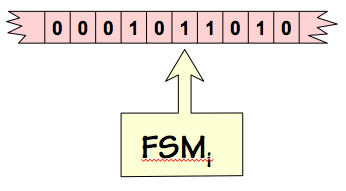Turing Machine i
Indeed, we can usurp the enumeration of FSMs sketched in Section 13.1.1 to enumerate Turing Machines, using the convention that $TM_i$ consists of $FSM_i$ interfaced to an infinite tape. Of course, many of these machines make little sense, e.g. due to a mismatch between their number of inputs and outputs and the needs of the tape interface; for our conceptual experiments, however, we can tolerate such coding inefficiencies and simply consider these mismatches to be non-functional Turing Machines. Armed with this convention, the set of possible Turing Machines is enumerable: every possible Turing Machine is $TM_i$ for some finite nonnegative $i$.

### 13.2.1. About that Infinite Tape

The use of an infinite tape certainly marks the Turing machine as a conceptual rather than a practical tool and may provoke some skepticism in the practical-minded reader.

In fact our interest is in practical computations: ones that complete in a finite number of steps. Every such finite computation will visit at most a finite number of positions on the infinite tape: it will read finitely many positions as input, and write finitely many positions as output. So every finite computation -- every one of the practical computations in which we profess interest -- can be completed with a finite tape of some size. The difficulty that motivated us to consider Turing machines rather than FSMs is that we cannot put a finite bound on that size without restricting the set of functions that can be computed. A conceptually convenient way to relax that restriction is to simply make the storage (the tape) infinite.

There are plausible alternatives to this abstraction mechanism. We might, for example, replace each Turing machine $TM_i$ by a family of finite-memory Turing machines $TMF_i^S$ with varying values of $S$, a finite tape size. The statement that "computable function $f_i$ can be performed by Turing machine $TM_i$" would then become "function $f_i$ can be performed by $TMF_i^S$ for sufficiently large S. We choose to duck this complication by the conceptually simple notion of an infinite tape.

Do keep in mind, however, that every finite computation uses at most a finite portion of the tape: it will read and write at most some bounded region surrounding the initial tape head position.

TMs as Integer Functions

### 13.2.2. TMs as Integer Functions

In order to compare the computational power of Turing Machines with alternative models such as those mentioned in Section 13.1.4, it is useful to characterize the computation performed by a given TM as an integer function -- that is, a function taking an integer input and producing an integer output. To do so, we adopt a convention for encoding a bounded tape configuration -- a configuration containing finitely many 1s (other positions containing 0) -- as a nonnegative number.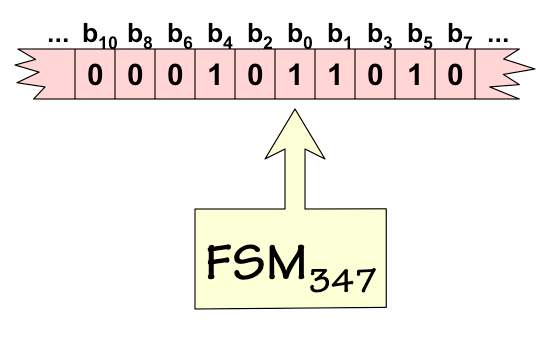TM 347 on tape 51
One possible such encoding is illustrated on the left. We take the binary digits stored on the infinite tape in a prescribed order to form a binary number whose low-order bit comes from the current head position, whose next bit is the position to the right of the head, whose third bit is from the position to the left of the head, then two positions to the right, and so on until all the (finitely many) 1 bits have been accounted for. The resulting binary number contains infinitely many leading 0s, but only finitely many 1s; hence its value is finite. This value represents the encoding of the tape configuration.TM as integer function
Armed with this convention, we can view each of our enumerable set of Turing Machines as computing an integer function. To compute the value of $y = TM_i[x]$, we mount an input tape containing the configuration $x$ on the machine, start the machine, let it run until it halts, and read the final tape configuration as the integer value $y$. Of course, in some cases the Turing Machine might run forever without halting, a problem we will return to shortly; for the present, we simply view the value of such non-terminating computations as undefined. We thus recharacterize TMs as computing partial functions that may not be defined for every possible integer argument.
Functions as Expressive Power

## 13.3. Functions as Expressive Power

Mathematicians studying these early models of computation used the set of integer functions computable by each model as a measure of that model's expressive power -- the idea being that if a given function (say, square root) could be expressed in one model but not in another, the latter model is less powerful in some mathematically significant sense. There ensued a period during which the set of integer functions computable by each of the proposed models was actively studied, partly motivated the desire to "rank" the proposed models by expressive power.

It is worth noting that the set of integer functions in question can be generalized in various ways. Functions of multiple integer arguments can be encoded using integer pairing schemes -- for example, representing the pair $(x,y)$ of arguments to $f(x,y)$ as a single integer whose even bits come from $x$ and whose odd bits come from $y$. Similarly, representation tricks like those sketched in Chapter 3 can allow symbols, approximations of real numbers, and other enumerable data types to be represented among the integer arguments and return values of the functions being considered. These coding techniques allow us to represent a very wide range of useful computations using integer functions -- in fact, using single-argument functions defined only on natural numbers (nonnegative integers).

An N-way tie!

### 13.3.1. An N-way tie!

The remarkable result of this attempt to identify the most expressive of the candidate models of computation, by the metric of expressibility of integer functions, was that each of the models studied could express exactly the same set of integer funtions. The equivalance of the models by this metric was proven, by showing systematic techniques for translating implementations in one model to equivalent implementations in another. For example, there is a systematic algorithm to derive from the description of any Turing machine a Lambda expression that performs precisely the same computation, implying that the expressive power of Lambda expressions is at least that of Turing machines. Moreover, there is an algorith for translating any Lambda expression to a Turing machine that performs the same computation, implying that Lambda expressions are no more expressive than Turing machines. This same set of integer functions may be implemented in many other computation schemes, a set including those mentioned in Section 13.1.4 and countless others, among them most modern computer languages.
Church's Thesis

### 13.3.2. Church's Thesis

The discovery that the same set of integer functions is computable by every computation scheme of sufficient power that was studied strongly suggests that that set of functions has a significance that goes beyond the properties of any given model of computation. Indeed, it suggests that there is an identifiable set of computable functions that can be implemented by any sufficiently powerful computation scheme, and functions outside this set cannot be computed by any machine we might implement now or in the future.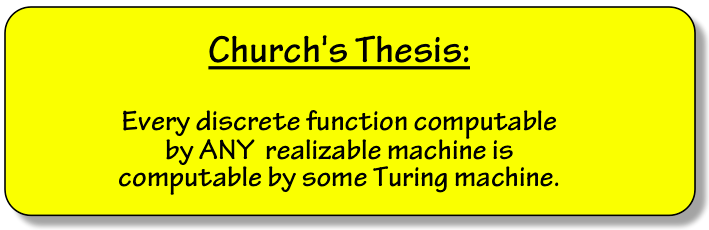This conjecture, which is widely believed and supported by countless failures to demonstrate the effective computation of integer functions that cannot be computed by Turing Machines, is often referred to as Church's Thesis in honor of Alonso Church. We cannot prove Church's thesis, due in part to the unbounded nature of the universe of "realizable machines" it covers; but we, and the Computer Science community generally, accept it as axiomatic.
Computable Functions

### 13.3.3. Computable Functions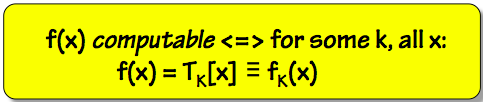Computable Functions
Indeed, we may use Church's thesis to define the set of computable functions as that set of functions computable by some Turing Machine. Given our ability to enumerate Turing Machines, it follows that we can enumerate Computable Functions in an infinite list. We might expand this list into an infinite two-dimensional spreadsheet, in order to represent the behavior of each computable function with every possible value for its single argument: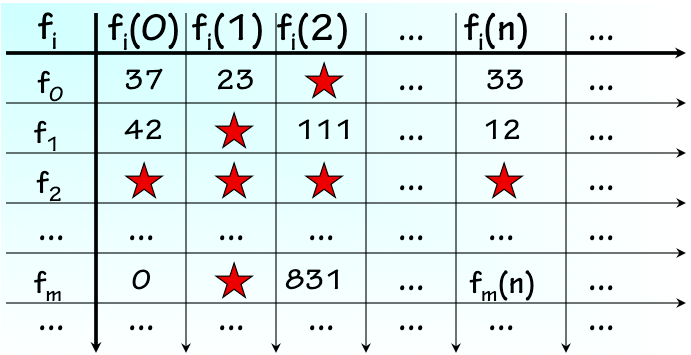Enumeration of Computable Functions
Note that this diagram is the upper-left corner of a table that extends to infinity in the right and downward directions. The computable functions (equivalently, Turing Machines) are itemized along the vertical axis, and succesive values of the single argument (equivalently, bounded tape configuration) along the horizontal axis. In each cell at the intesection of column $n$ and row $m$ we enter the result of computing $f_m(n)$, or (eqivalently) the final tape configuration after $TM_m$ is run on tape $n$.

In order to deal with the occasional case where $TM_m$ applied to tape $n$ computes forever without halting, we place a red star in cells corresponding to undefined values.

Of course, we cannot build this table any more than we can write down our infinite list of FSMs or Turing machines. Not only is the table infinite, but (as we will see shortly) there is no effective way to tell which cells should contain red stars. Nonetheless, the contents of each cell in the table is mathematically well defined, despite our practical inability to write it down.

We might ask whether the set of computable functions represented in this table includes every function defined on natural numbers -- i.e., whether the set of computable functions includes all possible functions of nonnegative integers. Unfortunately, this cannot be the case: the set of all functions defined on natural numbers is uncountably infinite, implying that there is no systematic way to enumerate them along the vertical axis of our spreadsheet. There are simply too many functions to enumerate; hence most functions are not represented on our list, and consequently not among the set of functions we have identified as computable.

The Halting Problem

### 13.3.4. The Halting Problem

Given the range of useful functions performed by modern computers, each demonstrably implemented using computable functions, it may be surprising to the uninitiated reader that the majority of well-defined functions are in fact uncomputable. In this section we demonstrate an example of an uncomputable function.

Consider the problem of determining whether Turing Machine $k$ will halt given input tape $j$ -- equivalently, whether the $k^{th}$ computable function applied to the argument $j$, $f_k(j)$, is well defined. It would be useful to have an effective implementation of this function, for example, if we were building a program to answer questions about values in the infinite table of the previous section.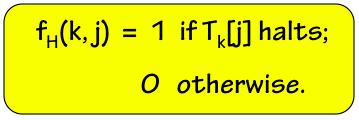The Halting Function
We can easily characterize this computation as a function of two integer arguments, as shown on the right: the halting function $f_H(k,j)$ returns 1 or 0 for every pair of arguments $k$ and $j$, and the value returned reflects whether $TM_k$ halts given tape $j$. In fact, a simple but head-spinning argument shows that this function is uncomputable.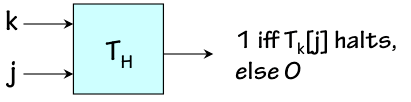Halting TM
If $f_H$ is computable, it can be computed by some Turing Machine; lets call this machine $TM_H$. $TM_H$ takes inputs $k$ and $j$, and produces (on its output tape) a value 1 or 0 indicating whether Turing Machine $TM_k$ halts given tape $j$.

If $TM_H$ performs a computable function, we can use it as a component to implement other computable functions. In particular, if $TM_H$ exists, there exists another Turing Machine $TM_N$ constructed as follows: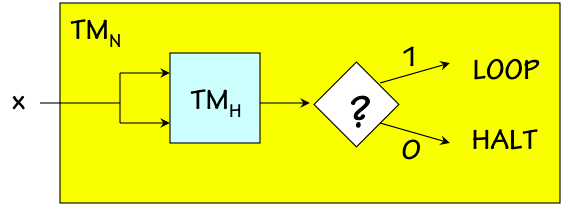This peculiar machine takes a single argument $x$, and uses the halting TM to determine whether $TM_x$ halts given the input $x$. If $TM_x[x]$ halts, $TM_N[x]$ loops forever; otherwise, $TM_N[x]$ halts and returns the value 0.

If $TM_H$ exists (i.e., is a computable function), then $TM_N$ must exist as well; we can argue informally by Church's thesis since a machine behaving like $TM_N$ seems clearly buildable if $TM_H$ is available as a component. If pressed, however, we could avoid the dependence on Church's thesis and provide details of the construction of $TM_N$ given the implementation details of $TM_H$.

However, the existance of $TM_N$ leads to a contradiction. Consider providing $TM_N$ with its own index -- $N$ -- as an argument. Will this computation halt?

By our construction of $TM_N$, $TM_N[N]$ must loop forever if Turing Machine $N$ halts with argument $N$ -- i.e, if $TM_N[N]$ halts. Thus $TM_N[N]$ halts implies $TM_N[N]$ loops, a contradiction. Similarly, $TM_N[N]$ must halt if $TM_N[N]$ loops forever, another contradiction. Any possible behavior of $TM_N$ for the input $N$ thus violates its specification, a specification that it provably obeys assuming its $TM_H$ component behaves as specified. Hence we must conclude that there is no Turing Machine $TM_H$.

This terse sketch of the uncomputabilty of the halting problem is genuinely confusing to those seeing it for the first time. In fact, it is an example of a diagonal argument technique pioneered by Cantor.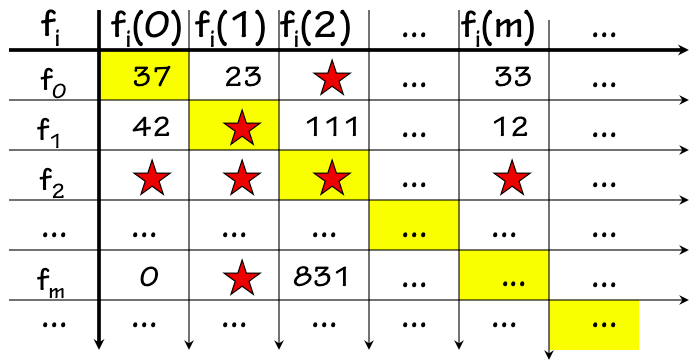The idea is to take a two-dimensional infinite table such as our spreadsheet of computable function behaviors and to synthesize a new row that is guaranteed to differ from each of the existing rows in at least one place, namely along the table's diagonal. The newly synthesized row cannot be identical to any existing row of the table, as it differs from each row in at least one column.

In particular, the function computed by $TM_N$ will differ from each $TM_i$ represented in our table for the argument $i$; it is designed to behave differently from each $TM$ by using the halting function to dictate behaviors to avoid along the shaded diagonal entries. The new Turing Machine $TM_N$ will behave differently from every $TM_x$ given argument $x$, halting if $TM_x(x)$ loops or looping if $TM_x(x)$ halts. Thus $TM_N$ will differ from every Turing Machine represented in our table, which comprise all of the computable functions. $TM_N$ consequently cannot be a computable function. Since it can evidently be constructed given $TM_H$, the halting function cannot be computed either.

Universal Turing Machines

## 13.4. Universal Turing Machines

Given the correspondence between computable functions and Turing Machines, we face the prospect of a different Turing Machine design for every computable function we might wish to compute. Indeed, we might have different special-purpose physical machines to multiply, take square roots, or compute payroll taxes: each machine would consist of an application-specific FSM intefaced to an infinite tape. Each Turing Machine is a special-purposecomputer, whose application-specific behavior is wired into the design of its FSM.

It seems clearly possible, however, to cut down on our inventory of special-purpose TMs by designing TMs capable of performing multiple functions. A single Turing Machine could certainly be capable of multiplying and taking square roots, depending on the value of an input devoted to specifying which function to perform. Indeed, a little thought will convince us that if functions $f_1(x)$ and $f_2(x)$ are both computable, then the function \begin{equation} f_{1,2}(x,y) = \begin{cases} f_1(x), & \mbox{if } y = 1 \\ f_2(x), & \mbox{if } y = 2 \end{cases} \end{equation} is also computable. Our Church's Thesis insight convinces us that, given physical machines that compute $f_1$ and $f_2$, we could build a new machine that computes $f_1$ or $f_2$ depending on the value of a new input. More formally, we could (with some effort) demonstrate an algorithm that takes the state transition diagrams of FSMs for two arbitrary Turing Machines $TM_i$ and $TM_j$, and constructs the diagram for a new $TM_{i,j}$ capable of behaving like either $TM_i$ or $TM_j$ depending on an input value.

If a single Turing Machine might evaluate any of several computable functions, is it possible that a single Turing Machine might be capable of evaluating every computable function? We can formalize this question by formulating a simple definition of a universal computable function $U(k,j)$ as follows: $$U(k, j) = TM_k(j)$$ This Universal function takes two arguments, one identifying an arbitrary Turing Machine (hence an arbitrary computable function) and a second identifying an input tape configuration (hence an argument to that function). It returns a value that is precisely the value returned by the specified Turing Machine operating on the given tape: the computation $U(k,j)$ involves emulating the behavior of $TM_k$ given tape $j$.

The existence of a TM capable of evaluating every computable function now amounts to the question of whether $U(k,j)$ is a computable function: if it is, then by our definition it is computed by some Turing Machine, and that machine is universal in the sense that it can compute every function computable by every Turing Machine. Given our discovery that the majority of functions are (sadly) not computable, we might be pessimistic about the computability of $U(k,j)$. Remarkably, however, $U(k,j)$ is computable.

There are in fact an infinite number of Turing Machines that are universal in the above sense. Each universal machine takes input that comprises two segments:

• A program specifying an algorithm to be executed (argument $k$ of $U(k,j)$); and
• Some data that serves as input to the specified algorithm (argument $j$ of $U(k,j)$).
The universal machine applies the specified algorithm to the specified data, generally by simulating the behavior of a machine executing the specified algorithm. It uses the program input to dictate a sequence of operations to execute on the specified input data, emulating the behavior of some particular Turing machine given that data. Of course, that same sequence of computational steps could be represented in other forms, such as a program in a contemporary programming language.
Turing Universality

### 13.4.1. Turing Universality

Universal Turing Machines have the remarkable property that they can mimic the behavior of any other machine, including that of other universal Turing machines, at least by the measure of the functions they can compute. That measure seems to capture their computational scope, although it ignores dimensions of their power like performance and details of I/O behavior. A universal Turing machine without audio output hardware cannot play audio, for example, although it can compute a stream of bits that represents a waveform to be played.

Of course, not every Turing machine is universal; the Unary Incrementor of Section 13.2, for example, cannot mimic the computational behavior of an arbitrary Turing machine. The distinction between machines that are universal in the Turing sense and those that are not has emerged as a basic threshold test of computational power. It is applicable not only to Turing machines, but to arbitrary models of computation -- including computer designs and programming languages. A programmable device or language that is Turing Universal has the power to execute any computable function, given the right program. By this standard, it is at the limit of computational power.

Interpretation

### 13.4.2. Interpretation

The ability of a universal machine to mimic the behavior of another machine is the basis for a powerful engineering abstraction mechanism, one that underlies the field of Computer Science. That mechanism is the interpretation of a coded algorithm -- a program -- to effect the execution of that algorithm. Imagine, for example, we have a simple universal machine $M1$ which is easy to manufacture but difficult to program due to its primitive nature. The language $L1$ accepted by $M1$ for its programs passes the universality test, but may require every operation to be expressed in terms of single-bit NANDs rather than (say) arithmetic operations.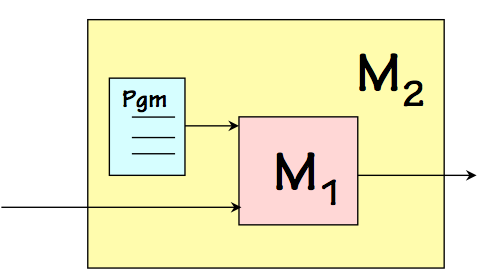Interpretation
We might improve its programmability by designing a more programmable machine, $M2$, and writing and writing a single program in $M1$ that simulates the behavior of $M2$. That program -- an interpreter for the language of $M2$ written in the language of $M1$ might be an engineering challenge, but it is the only program that needs to be written in the maligned $L1$ language. We can then package the interpreter with an M1 machine, resulting in a machine that behaves like the more humane $M2$.

Many successful computers have been designed following this strategy. Their user-visible functionality is that of a machine that executes high-level operations of interest to programmers, but their hardware executes low-level operations coded as firmware embedded in read-only memory hidden within the machine. This internal program is often called microcode, to distinguish it from the programming interface advertised for the machine.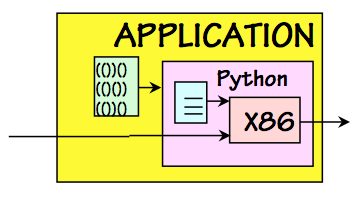Layers of Interpretation
In modern computer systems, we often find multiple layers of interpretation operating simultaneously. The diagram on the left shows an application written in Python, a language implemented using an interpreter written in the machine language provide by its host machine (say, the Intel X86 instruction set). That X86 machine language is itself interpreted by a hardware CPU. Indeed, a closer look might reveal more interpretive layers: the application may itself be an interpreter (for example, a browser interpreting HTML); and the host machine may use microcode to implement its instruction set. We may view this implementation as a nest of virtual machines, each implemented using an internal lower-level machine and a programmed interpreter.Language Interfaces
An alternative view, shown on the right, highlights the languages that interface adjacent interpretive layers. The hardware interprets X86 machine language programs. The Python interpreter, coded as X86 instructions, interprets the Python language. The application, coded in Python, interprets its application-specific data.

This diagram emphasizes the separation of implementation technologies -- the interpreters -- from the languages that interface them to one another. The clarity of this separation between implementation and interface makes interpretation an extremely powerful tool for engineering abstraction.

Compilation

### 13.4.3. Compilation

The preceeding examples show interpretation as a mechanism for moving from one interface language to another -- for example, for executing Python programs on a machine that can execute X86 machine language. There is an alternative mechanism for this language-level mobility, also commonly used in modern systems, that is subtly different from interpretation: we refer to it as compilation. While interpretation involves emulating the behavior specified by an encoded algorithm, compilation involves translating an algorithm from one language to another.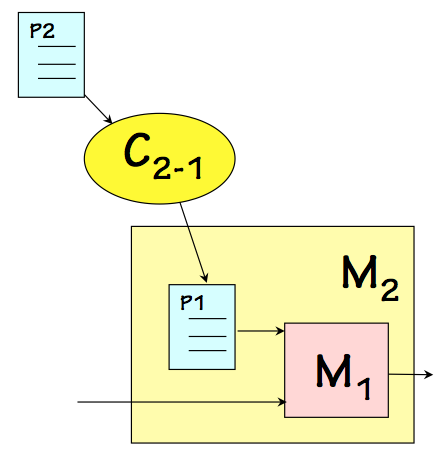Compilation
Suppose again that you have a universal machine $M1$ whose programming language $L1$ is too low level to be useful. You prefer the language $L2$ of some hypothetical machine $M2$. Rather than programming an interpreter for $L2$ in the language $L1$ as before, you might write a separate program $C_{2-1}$ that translates programs written in $L2$ to equivalent programs in $L1$. Such a program is called a compiler. Given such a compiler, a programmer who wants to run a program $P2$ written in the humane language $L2$ can simply pass it to the $C_{2-1}$ compiler once to generate the equivalent program $P1$, which can be run on $M1$ arbitrarily many times.

Like interpretation, compilation offers a way to write programs in a language other than that accepted by the target machine. However, there are some important practical differences between the two approaches:

• what: Interpreters and compilers both take a progrogram as input; however, the interpreter produces behavior as a result, while the compiler produces another program.
• where: The interpreter runs on the target machine (hence must be coded in the language of that machine); the compiler can run on any machine, and may hence be coded in an arbitrary language.
• how often: Interpretation is a mechanism for execution, and takes place whenever the interpreted program is executed. Compilation is a single translation step that yields a program that can be run (without re-compilation) many times. For this reason, compilation is typically more time-efficient for programs that are executed many times.
• when: Interpreted code makes implementation choices while the program is being executed (at "run time"); compilers make certain choices during the preliminary translation process ("compile time").
Compilation and interpretation are both important tools that belong in the repertoire of every engineer. We will see many examples of their use in subsequent chapters.
Context: Foundations of Mathematics

## 13.5. Context: Foundations of Mathematics

The foundational ideas of computer science discussed in this Chapter arose during a period in which a number of eminent mathematicians were preocccupied with the project of putting the field of mathematics itself on a logically sound conceptual basis. We sketch some landmarks of this history below:
Set theory as a basis for Mathematics:
During the $19^{th}$ century, the idea emerged that all of mathematics could be based on set theory. This is not the set theory involving sets of apples and oranges that many of us encountered in our early education; rather, the pure mathemeticians espousing this view wanted to avoid postulating arbitrary concrete artifacts (like apples -- or for that matter numbers) and construct all of mathematics from a set of basic principles, namely the handful of axioms defining set theory.

What concrete things can one construct from the axioms of set theory and nothing else? Well, we can construct the empty set. This concrete artifact gives some meaning to the notion of zero, the number of elements in the empty set. Given this first concrete artifact, you can make a set whose single element is the empty set, giving meaning to $1$ as a concrete concept. Following this pattern, one can build $2$, $3$, and the entire set of natural numbers. Additional conceptual bootstrapping steps lead to rationals, reals, and complex numbers; eventually, it can provide the foundations for calculus, complex analysis, topology, and the other topics studied in mathematics departments of contemporary universities.

At about the start of the $20^{th}$ century, Bertrand Russel observed that a cavalier approach to set theory can lead to trouble, in the form of logical inconsistencies in the theory being developed as the basis of all mathematics. Logical theories are frail, in that introduction of a single inconsistency -- say, $5 = 6$ -- gives them the power to prove any formula independent of its validity. For a formula $X$, an inconsistent logic could be used to prove both $X$ and not $X$, defeating its usefulness in distinguishing valid from invalid formulae.

Russel's famous example of ill-formed sets involved the village barber who shaves every man who doesn't shave himself -- a homey proxy for a set containing all sets not contaning themeselves. Does such a set contain itself? Either answer leads to a contradiction, revealing an inconsistency in set theory.

Other Russel folklore includes his public assertion that any inconsistency allows every formula to be proved, prompting a challenge from the audience for Russel to prove himself the Pope given the assertion that $5=6$. The story has Russel accept and elegantly fulfill that challenge.

Hilbert's Program:
While the set theoretic pitfall observed by Russell was fairly easy to sidestep, it raised an important issue: how can we be sure that all of mathematics is in fact on a sound basis? It would be disasterous for centuries of mathematicians to prove new results, only to find that each of these results could be disproved as well due to an undetected inconsistency in the underlying logic assumed.

A number of mathematicians, famously including David Hilbert, undertook a program to assure mathematics a provably sound basis. Their goal was to establish an underlying logic which was simultaneously

• consistent, meaning that only valid formulae could be proved; and
• complete, meaning that every valid formula can be proved.

Of course, the desired logic would first be used to prove its own consistency and completeness, assuring mathematics a future as the undisputed basis for rational distinction between truth and falsehood.

Gödel's Incompletness Theorm:
In 1931, the Austrian mathematician Kurt Gödel shocked world of mathematics and shattered the lofty goals of Hilbert's program. Gödel showed that any logical theory having the power to deal with arithmetic contains a formula equivalent to the English sentence "this statement can't be proved". A moment's reflection on the logical validity of this sentence reveals a problem: if it can be proved, the statement is false; hence the theory is inconsistent, allowing the proof of a false statement. If on the other hand it cannot be proved, the statement is true and the theory incomplete: there is a valid formula that cannot be proved.

In this monumental work, Gödel pioneered many of the techniques sketched in this Chapter -- including enumeration (often called Gödel numbering in his honor) and diagonalization. It establishes that every sound logical theory of sufficient power contains unprovable formulae: there are bound to be true statements that cannot be proven.

Gödel used the power of mathematics to circumscribe the limits to that power itself.

Computability:
In the wake of Gödel's bombshell, Turing, Church, and others showed that not all well defined functions are computable. Indeed, Turing showed moreover that not all numbers are computable by an intuitively appealing measure of computability. This succession of negative results during the 1930's challenged the field of mathematics as the arbiter of rational truth.

Universality:
Interspersed among these demonstrations of the limits of mathematics was the discovery that the tiny minority of functions that are computable comprises a remarkably rich array of useful computations. Of particular interest -- at the time, in a theoretical sense -- was the discovery that the universal function of Section 13.4 is in theory a computable function.

General-purpose Computing:
The universal Turing machine remained a conceptual experiment for a decade, until researchers began exploring the construction of practical machines based on the idea. Conspicuous among these was John von Neumann, whose landmark 1945 paper sketched the architecture of a machine design that has become recognised as the ancestor of modern general-purpose computers.

Since the articulation of the von Neumann architecture, many profound conceptual and technology developments have driven the computer revolution. These include core memory, the transistor, integrated circuits, CMOS, and many others. But the basic plan for a stored-program general purpose computer, detailed in the next Chapter, has clear roots in von Neumann's vision and the universal function on which it is based.

Chapter Summary

## 13.6. Chapter Summary

The conceptual tools discussed in this Chapter unleash a powerful new engineering abstraction, one that enables engineering to be applied to a new and richer universe of structures. The key is that we can extract the information that defines the logical structure of a machine, and manipulate that information -- a block of 1s and 0s -- rather than manipulating the machine itself. The concept of interpretation allows us to move from a structural description to a behavioral one, freeing us from the need to represent each behavior by physical systems exhibiting that behavior.

Imagine the prospect of building a machine whose input is some physical machine that performs a computation, and whose output is a different physical machine performing the same computation but slightly faster. This "machine improver" machine would have to dissect the input machine, analyze its behavior, and assemble the output machine from some inventory of parts. The process would be unimagineably complex and clearly impractical.

However, computers perform such transformations commonly, by dealing with coded descriptions -- algorithms -- rather than with physical machines whose behavior the algorithms represent. It is the liberation of algorithms from the physical devices in which they are embodied that promotes behaviors -- coded as algorithms -- to become themselves objects of engineering.